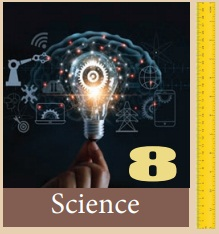Home | | Science 8th Std | Measurement

# Measurement

After the completion of this lesson, students will be able to: * understand fundamental quantities and units. * explain the system of units and measurements. * analyse the different system of units. * know about temperature, amount of substance, electric current and luminous intensity. * explore the knowledge of accuracy in measurements. * differentiate plane angle and solid angle. * solve problems related to measurement.

UNIT 1

MEASUREMENTLearning Objectives

After the completion of this lesson, students will be able to:

* understand fundamental quantities and units.

* explain the system of units and measurements.

* analyse the different system of units.

* know about temperature, amount of substance, electric current and luminous intensity.

* explore the knowledge of accuracy in measurements.

* differentiate plane angle and solid angle.

* solve problems related to measurement.Introduction

Physics is the study of nature and natural phenomena. Physics is considered as the base of all science subjects. It is based on experimental observations. The principles and observations allow us to develop a deeper understanding of nature. Scientific theories are valid, only if they are confirmed through various experiments. Theories in physics use many physical quantities that have to be measured.

Measurement is the base of all scientific studies and experimentations. It plays a vital role in our daily life. It is the process of finding an unknown physical quantity by using a standard quantity. In this lesson, we will study about measurement in detail. We will also study about accuracy and precision, approximation and rounding off.

Activity 1

Measure the length and breadth of your science book using a ruler (scale) and 8 compare your value with those of your friends.Students arc asked to measure the length and breadth of their science book using a ruler (scale) and compare their measurement with those of their friends.

Aim : To determine the length and breadth of science book and to understand magnitude and unit.

Apparatus required: Science book and ruler (scale)

Tabular column:

Name of the object : Science textbook

Length (cm) : 25

Inference :

The length of science book  l = 25 cm

The breath of science book b = 21 cm

Here, 25, 21 is the magnitude (number value) cm is the unit.

Tags : Chapter 1 | 8th Science , 8th Science : Chapter 1 : Measurement
Study Material, Lecturing Notes, Assignment, Reference, Wiki description explanation, brief detail
8th Science : Chapter 1 : Measurement : Measurement | Chapter 1 | 8th Science